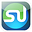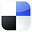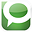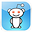# Using the Confidence Interval in Excel To Find Customer Preferences

This article will show you exactly how to calculate a 95% Confidence Interval in Excel of the proportion of customers on a commercial web site who prefer to pay with a credit card instead of Paypal. The 95% Confidence Interval is the interval for which we are 95% sure that the true proportion of credit card-preferring customers lies within.

The larger the sample size, the more accurate and smaller the confidence interval will be. We are taking a random sample of 1,000 Internet shoppers on this e-commerce web site.

The advantages of statistical analysis in Excel to solve business statistics problems is that most problems can be solved in just one or two steps and there is no more need to look anything up on Normal Distribution tables.

The 95% Confidence Interval calculated here is a Confidence Interval for Proportion, not Means. A Confidence Interval for Means uses an entirely different set of statistical formulas. Another article in this blog shows exactly how to calculate a Confidence Interval Means. Here is the problem:

Problem: A random sample of 1,000 Internet shoppers on one web site was taken. 70% preferred to pay with a credit card. 30% preferred to pay with PayPal. Determine the 95% Confidence Interval for the proportion of the general population of shoppers on that web site that prefers to pay with a credit card.

Before we begin solving this problem, we need to know about creating Confidence Intervals in Excel. Here is a brief lesson:

Confidence Interval of a Population Proportion

Creating a Confidence Interval for a population's proportion is very similar to creating a Confidence Interval for a population's mean. The only real difference is how the standard error is calculated. Everything else is the same.

First, the difference between using sampling to estimate a population mean and using sampling to estimate a population proportion will be explained below:

Mean Sampling vs. Proportion Sampling

What determines whether a mean is being estimated or a proportion is being estimated is the number of possible outcomes of each sample taken.

Proportion samples have only two possible outcomes
. For example, if you are comparing the proportion of click-through visitors in two different PPC ads who converted (purchased), each sample has only two possible values; the visitor sampled either converted (purchased) or they didn't.

Mean samples have multiple possible outcomes
. For example, if you are comparing the mean sales of salespeople in two different cities, each salesperson's sales sampled can have numerous values.

Below is a description of how to calculate a Confidence Interval for a population's proportion. Note that everything is almost the same as the calculation of the Confidence Interval for a mean, except sample standard error.

Levels of Confidence and Significance

Level of Significance
, α ("alpha"), equals the maximum allowed percent of error. If the maximum allowed error is 5%, then α = 0.05.

Level of Confidence
is selected by the user. A 95% Level is the most common. A 95% Confidence Level would correspond to a 95% Confidence Interval of the Proportion.

This would state that the actual population Proportion has a 95% probability of lying within the calculated interval. A 95% Confidence Level corresponds to a 5% Level of Significance, or α = 0.05. The Confidence Level therefore equals 1 - α.

Population Proportion vs. Sample Proportion

Population Proportion
= µp = p (This is what we are trying to estimate)

Sample Proportion
= pavg

Standard Deviation and Standard Error

Standard Deviation
is not calculated during the creation of Confidence Interval for a population proportion.

Standard Error
is an estimate of population Standard Deviation from data taken from a sample. Sample Standard Error will be an estimate taken from the sample proportion, pavg, and sample size, n. This is the major difference between calculating a Confidence Interval for a proportion and for a mean. Binomial distribution rules apply to proportions because a proportion sample has only two possible outcomes, just like a binomial variable.

Sample Standard Error of a Proportion
= σpavg = SQRT(p * q / n) ≈ spavg

= spavg = SQRT ( pavg * qavg / n )

p
= Population proportion - This is the unknown that will be estimated with a Confidence Interval

q = 1 - p

n
= Sample size

p
avg = Sample proportion

q
avg = 1 - pavg

Region of Certainty vs. Region of Uncertainty

Region of Certainty
is the area under the Normal curve that corresponds to the required Level of Confidence. If a 95% percent Level of Confidence is required, then the Region of Certainty will contain 95% of the area under the Normal curve. The outer boundaries of the Region of Certainty will be the outer boundaries of the Confidence Interval.

The Region of Certainty, and therefore the Confidence Interval, will be centered about the mean. Half of the Confidence Interval is on one side of the mean and half on the other side.

Region of Uncertainty
is the area under the Normal curve that is outside of the Region of Certainty. Half of the Region of Uncertainty will exist in the right outer tail of the Normal curve and the other half in the left outer tail. This is similar to the concept of the "two-tailed test" that is used in Hypothesis testing in further sections of this course. The concepts of one and two-tailed testing are not used when calculating Confidence Intervals. Just remember that the Region of Certainty, and therefore the Confidence Interval, are always centered about the mean on the Normal curve.

Relationship Between Region of Certainty, Uncertainty, and Alpha

The Region of Uncertainty corresponds to α ("alpha"). If α = 0.05, then that Region of Uncertainty contains 5% of the area under the Normal curve. Half of that area (2.5%) is in each outer tail. The 95% area centered about the mean will be the Region of Certainty. The outer boundaries of this Region of Certainty will be the outer boundaries of the 95% Confidence Interval.

The Level of Confidence is 95% and the Level of Significance, or maximum error allowed, is 5%.

Z Score

Z Score is the number of Standard Errors from the mean to outer right boundary of the Region of Certainty (and therefore to the outer right boundary of the Confidence Interval).

Standard Errors are used and not Standard Deviations because sample data is being used to calculate the Confidence Interval.

Z Score is calculated by the following Excel function:

Z Score(1-α) = NORMSINV (1 - α/2) - This will be discussed shortly.

Excel Functions Used When Calculating Confidence Interval for a Population Proportion

Note that Excel functions STDEV and AVERAGE are not used when working with proportions. The CONFIDENCE function is not used either.

Excel functions that are used are:

COUNT
(Highlighted block of cells) = Sample size = n

This counts the number of cells in highlighted block

NORMSINV
(1 - α/2) = Z Score(1 - α)

Number of Standard Errors from mean to boundary of Confidence Interval. Note that (1 - α/2)

= the entire area in the Normal curve to the left of outer right boundary of the Region of Certainty, or Confidence Interval. This includes the entire Region of Certainty and the half of the Region of Uncertainty that exists in the left tail.

For example:

Level of Confidence = 95% for a 95% Confidence Interval

Level of Significance = 5% (α = 0.05)

1 - α = 0.95 = 95%

Z Score95% = NORMSINV (1 - α/2) = NORMSINV (1 - .05/2) = NORMSINV(1 - 0.025)

Z Score95% = NORMSINV (0.975) = 1.96

The outer right boundary of the 95% Confidence Interval, and the Region of Certainty, is 1.96 Standard Errors from the mean. The left boundary is the same distance from the mean because the Confidence Interval is centered about the mean.

Formula for Calculating Confidence Interval Boundaries from Sample Data for a Population Proportion

Confidence Interval Boundaries = Sample proportion +/- Z Score(1-α) * Sample Standard Error

Confidence Interval Boundaries = pavg +/- Z Score(1-α) * spavg

Sample Proportion = pavg

Z Score(1 - α) = NORMSINV (1 - α/2)

Sample Standard Error of a Proportion = σpavgspavg = SQRT ( pavg * qavg / n )

Sample size = n = COUNT (Highlighted block of cells containing samples)

Confidence Interval Boundaries = pavg +/- Z Score(1-α) * spavg

***************************************************
Before we use any statistical test that relies upon the underlying population data (from which samples are drawn) to be normally distributed, we must first test the data for normality. The Confidence Interval is an interval in the Normal curve. This requires that the underlying population data be Normally distributed.
***************************************************

Always Test for Normality First

Normality tests should be performed on the independent random sample of Internet shoppers. This blog has numerous articles about how to perform normality testing and nonparametric testing if the data is not normally distributed.

***************************************************

Now we're ready to solve the problem and create a Confidence Interval in Excel.

Problem: Determine Confidence Interval of Internet Shoppers Who Prefer to Pay By Credit Card Based Upon Sample Data

A random sample of 1,000 Internet shoppers on one web site was taken. 70% preferred to pay with a credit card. 30% preferred to pay with PayPal. Determine the 95% Confidence Interval for the proportion of the general population of Internet shoppers on that web site that prefers to pay with a credit card.

Level of Confidence = 95% = 1 - α

Level of Significance = α = 0.05

Sample Size = n = 1,000

Sample Proportion = pavg = 0.70

qavg = 1 - pavg = 0.30

Sample Standard Error of a Proportion = σpavgspavg = SQRT ( pavg * qavg  / n )

spavg = SQRT ( 0.70 * 0.30 / 1,000 ) = 0.014

Z Score(1 - α) = Z Score95% = NORMSINV (1 - α/2)

= NORMSINV (1 - 0.025) = NORMSINV (0.975) = 1.96

Width of Half the Confidence Interval = Z Score(1-α) * spavg

= 1.96 * 0.014 = 0.0274

Confidence Interval Boundaries = pavg  +/- Z Score(1-α) * spavg

= 0.70 +/- (1.96) * (0.014)

0.70 +/- 0.0274 = 0.6726 to 0.7274 = 67.26% to 72.74%

We can be 95% certain that the percentage of Internet shoppers on this e-commerce web site who prefer to pay with a credit card instead of Paypal is between 67.26% and 72.74%.

Click Image To See Larger Version

*****************************************

Confidence Interval in Excel To Find Customer Preferences

If You Like This, Then Share It...Excel Master Series Blog Directory

Statistical Topics and Articles In Each Topic

• Histograms in Excel
• Bar Chart in Excel
• Combinations & Permutations in Excel
• Normal Distribution in Excel
• t-Distribution in Excel
• Binomial Distribution in Excel
• z-Tests in Excel
• t-Tests in Excel
• Hypothesis Tests of Proportion in Excel
• Chi-Square Independence Tests in Excel
• Chi-Square Goodness-Of-Fit Tests in Excel
• F Tests in Excel
• Correlation in Excel
• Pearson Correlation in Excel
• Spearman Correlation in Excel
• Confidence Intervals in Excel
• Simple Linear Regression in Excel
• Multiple Linear Regression in Excel
• Logistic Regression in Excel
• Single-Factor ANOVA in Excel
• Two-Factor ANOVA With Replication in Excel
• Two-Factor ANOVA Without Replication in Excel
• Creating Interactive Graphs of Statistical Distributions in Excel
• Solving Problems With Other Distributions in Excel
• Optimization With Excel Solver
• Chi-Square Population Variance Test in Excel
• Analyzing Data With Pivot Tables
• SEO Functions in Excel
• Time Series Analysis in Excel